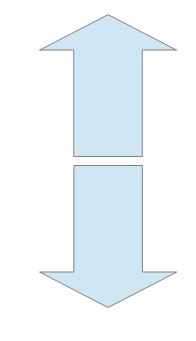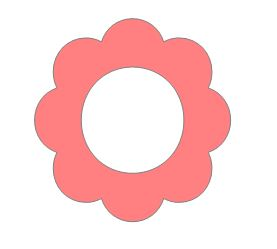# Geometry Review Step 3 Math

Which type of transformation is shown in this image?translation
reflection
rotation
Which type of transformation is shown in this image?reflection
translation
rotation

Which type of transformation is shown in this image?rotation
translation
reflection

Which types of symmetry does this shape have?both reflective and rotational
reflective
rotational

How many lines of reflective symmetry does this shape have?1
2
0

Create ratios and determine if these shapes are similar or not.2/4 and 3/6; Yes, they are similar.
2/3 and 4/6; No, they are not similar.
2/6 and 3/4; No, they are not similar.

Which of the following figures have rotational symmetry?
Equilateral Triangle and Parallelogram
Trapezoid and Rectangle
Equilateral and Isosceles Triangles
Rhombus and Scalene Triangle

True or False? The scale factor 1:3 is correct for the model given:
Nia created a 8 inch clay model of a horse. Later she enlarged the horse to 24 inches.
True
False

A rectangular prism consists of what figures?
1 rectangle and 4 triangles
2 squares and 4 rectangles
2 Circles and One Rectangle
A cylinder consists of what figures?
1 rectangle and 4 triangles
2 squares and 4 rectangles
2 Circles and One Rectangle

A rectangular pyramid consists of what figures?
1 rectangle and 4 triangles
2 squares and 4 rectangles
2 Circles and One Rectangle

The actual distance from Atlanta to Nashville is 250 miles. The scale on the map is 1/2 inch = 80 miles. Find the distance from Atlanta to Nashville on your map. Round your answer to the nearest tenth of an inch.

1.6 inches

On your map, the distance between Houston, TX and Abilene, TX is 5.5 cm. The scale on the map is 1 cm = 75 miles. Find the actual distance from Houston to Abilene.

412.5 miles

You measure the actual length of the garden house to be 24 feet. You need to draw a scale model of the layout of the house. You’ve been told the scale factor should be 2 inches = 10 feet. How long will you draw the length of the garden house?

4.8 inches

An artist has created a scale model of the Eiffel Tower. The scale for the model is 1 inch = 22 feet. If his model is 45 inches tall, how tall is the Eiffel Tower?

990 feet